# Precalculus : Algebraic Vectors and Parametric Equations

## Example Questions

1 2 3 5 Next →

### Example Question #41 : Algebraic Vectors And Parametric Equations

Find the parametric equations for a line parallel to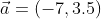and passing through the point (4, -3).

Possible Answers:

x = -3 + 3.5t

y = 4 - 7t

x = -3 - 3.5t

y = 4 + 7t

x = -7 + 4t

y = 3.5 - 3t

x = 4 - 7t

y = -3 + 3.5t

Correct answer:

x = 4 - 7t

y = -3 + 3.5t

Explanation:

A line through a point (x1,y1) that is parallel to the vector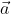= (a1, a2) has the following parametric equations, where t is any real number.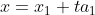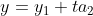Using the given vector and point, we get the following:

x = 4 - 7t

y = -3 + 3.5t

Each value of t creates a distinct (x, y) ordered pair. You can think of these points as representing positions of an object, and of t as representing time in seconds. Evaluating the parametric equations for a value of t gives us the coordinates of the position of the object after t seconds have passed.

### Example Question #42 : Algebraic Vectors And Parametric Equations

Write the parametric equation for the line y = -3x +1.5

Possible Answers:

x = -3t +1.5

y = -3t +1.5

x = t

y = 1.5t - 3

x = -3t +1.5

y = t

x = t

y = -3t +1.5

Correct answer:

x = t

y = -3t +1.5

Explanation:

In the equation y = -3x +1.5, x is the independent variable and y is the dependent variable. In a parametric equation, t is the independent variable, and x and y are both dependent variables.

Start by setting the independent variables x and t equal to one another, and then you can write two parametric equations in terms of t:

x = t

y = -3t +1.5

### Example Question #43 : Algebraic Vectors And Parametric Equations

Write the parametric equation for the line y = 5x - 3.

Possible Answers:

x = 5t - 3

y = 5t - 3

x = t

y = 5t - 3

x = t

y = t

x = 5t - 3

y = t

Correct answer:

x = t

y = 5t - 3

Explanation:

In the equation y = 5x - 3, x is the independent variable and y is the dependent variable. In a parametric equation, t is the independent variable, and x and y are both dependent variables.

Start by setting the independent variables x and t equal to one another, and then you can write two parametric equations in terms of t:

x = t

y = 5t - 3

### Example Question #44 : Algebraic Vectors And Parametric Equations

Write an equation in slope-intercept form of the line with the given parametric equations: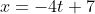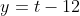Possible Answers: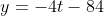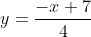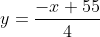Correct answer:Explanation:

Start by solving each parametric equation for t: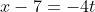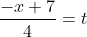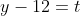Next, write an equation involving the expressions for t; since both are equal to t, we can set them equal to one another: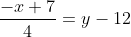Multiply both sides by the LCD, 4: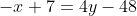Get y by itself to represent this as an equation in slope-intercept (y = mx + b) form: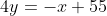### Example Question #45 : Algebraic Vectors And Parametric Equations

Write an equation in slope-intercept form of the line with the given parametric equations: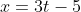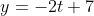Possible Answers: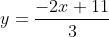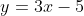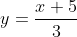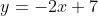Correct answer:Explanation:

Start by solving each parametric equation for t: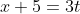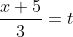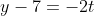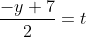Next, write an equation involving the expressions for t; since both are equal to t, we can set them equal to one another: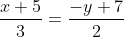Multiply both sides by the LCD, 6: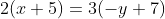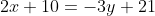Get y by itself to represent this as an equation in slope-intercept (y = mx + b) form: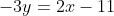### Example Question #46 : Algebraic Vectors And Parametric Equations

A football punter kicks a ball with an initial velocity of 40 ft/s at an angle of 29o to the horizontal. After 0.5 seconds, how far has the ball travelled horizontally and vertically?

Possible Answers: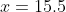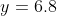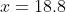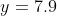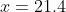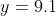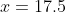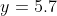Correct answer:Explanation:

To solve this problem, we need to know that the path of a projectile can be described with the following equations: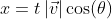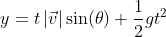In these equations, t is time and g is the acceleration due to gravity.

First, you need to write the position of the ball as a pair of parametric equations that define the path of the ball at anytime, t, in seconds: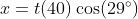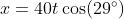As you set up the equation for y, use the value g = -32.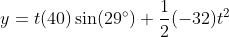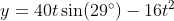Finally, find x and y when t = .05: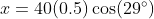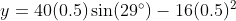Use a calculator to solve, making sure you are in degree mode:This means that after 0.5 seconds, the ball has travelled 17.5 feet horizontally and 5.7 feet vertically.

### Example Question #47 : Algebraic Vectors And Parametric Equations

A pitcher throws a fastball, and the batter swings and connects with the ball. If the ball has an initial velocity of 150 f/s and an angle of 20o about the horizontal, what parametric equations will model the motion of the ball? What height will the ball be at when it has travelled 400 feet horizontally?

Possible Answers: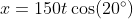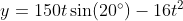Height of ball when it has travelled 400 ft is 14.38 feet above the groundHeight of ball when it has travelled 400 ft is 21.59 feet above the groundHeight of ball when it has travelled 400 ft is 16.65 feet above the groundHeight of ball when it has travelled 400 ft is 15.52 feet above the ground

Correct answer:Height of ball when it has travelled 400 ft is 16.65 feet above the ground

Explanation:

To solve this problem, we need to know that the path of a projectile can be described with the following equations:In these equations, t is time and g is the acceleration due to gravity.

First, you need to write the position of the ball as a pair of parametric equations that define the path of the ball at anytime, t, in seconds: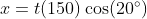As you set up the equation for y, use the value g = -32.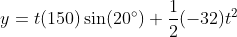Finally, find x and y when t = .05:To find the height that the ball will be at when it has travelled horizontally, plug 400 feet (horizontal distance) in for x.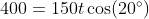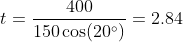This tells us that the ball has travelled 400 feet horizontally when 2.84 seconds have passed. Let's use the value of t = 2.84 and plug it into our equation for y to see how high the ball is at this time: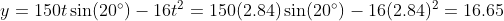This means that when the ball has travelled 400 feet horizontally, 2.84 seconds have passed, and the ball is 16.65 feet above the ground.

1 2 3 5 Next →

### All Precalculus Resources